# ISEE Middle Level Math : Data Analysis

## Example Questions

### Example Question #21 : Data Analysis

Find the range of this set of numbers: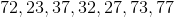Possible Answers: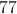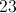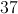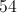Correct answer:Explanation:

The range of a data set is the difference between the smallest and largest numbers.

First, order the numbers from least to greatest: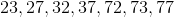Then, subtract the smallest number from the largest: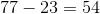### Example Question #14 : How To Find Range

Find the range of this set of numbers: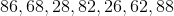Possible Answers: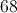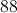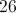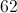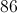Correct answer:Explanation:

First, order the numbers from least to greatest: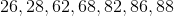Then, subtract the smallest number from the largest: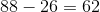### Example Question #21 : How To Find Range

Find the range of this set of numbers: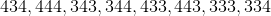Possible Answers: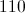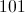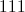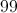Correct answer:Explanation:

First, order the numbers from least to greatest: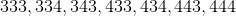Then, subtract the smallest from the largest number:### Example Question #22 : How To Find Range

Find the range in this set of numbers: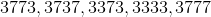Possible Answers: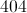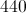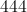Correct answer:Explanation:

First, order the numbers from least to greatest: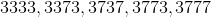Then, subtract the smallest from the largest number: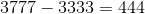### Example Question #23 : How To Find Range

What is the range of the following numbers?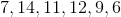Possible Answers: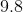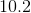Correct answer:Explanation:

The range is determined by subtracting the smallest value from the largest value.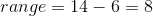### Example Question #24 : How To Find Range

Find the range in this set of numbers: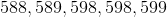Possible Answers: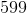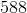Correct answer:Explanation:

First, order the numbers from least to greatest:Then, subtract the smallest number from the largest: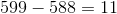Answer: The range is 11.

### Example Question #21 : How To Find Range

Find the range in this set of numbers: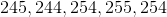Possible Answers: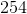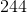Correct answer:Explanation:

First, order the numbers from least to greatest: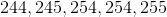Then, subtract the smallest number from the largest: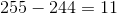Answer: The range is 11.

### Example Question #26 : How To Find Range

Find the range in this set of numbers: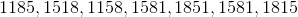Possible Answers: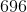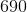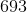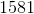Correct answer:Explanation:

First, order the numbers from least to greatest: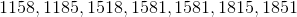Then, subtract the smallest number from the largest: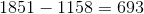Answer: The range is.

### Example Question #27 : How To Find Range

Find the range in this set of numbers: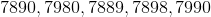Possible Answers: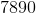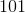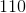Correct answer:Explanation:

First, arrange the numbers from least to greatest: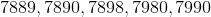Then, subtract the smallest number from the largest: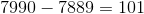Answer: The range is.

### Example Question #28 : How To Find Range

Find the range in this set of numbers: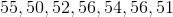Possible Answers:Correct answer:Explanation:

First, order the numbers from least to greatest: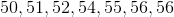Then, subtract the smallest number from the largest number: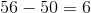Answer: The range is.

### All ISEE Middle Level Math Resources